Ex 4.2

Chapter 4 Class 8 Practical Geometry
Serial order wise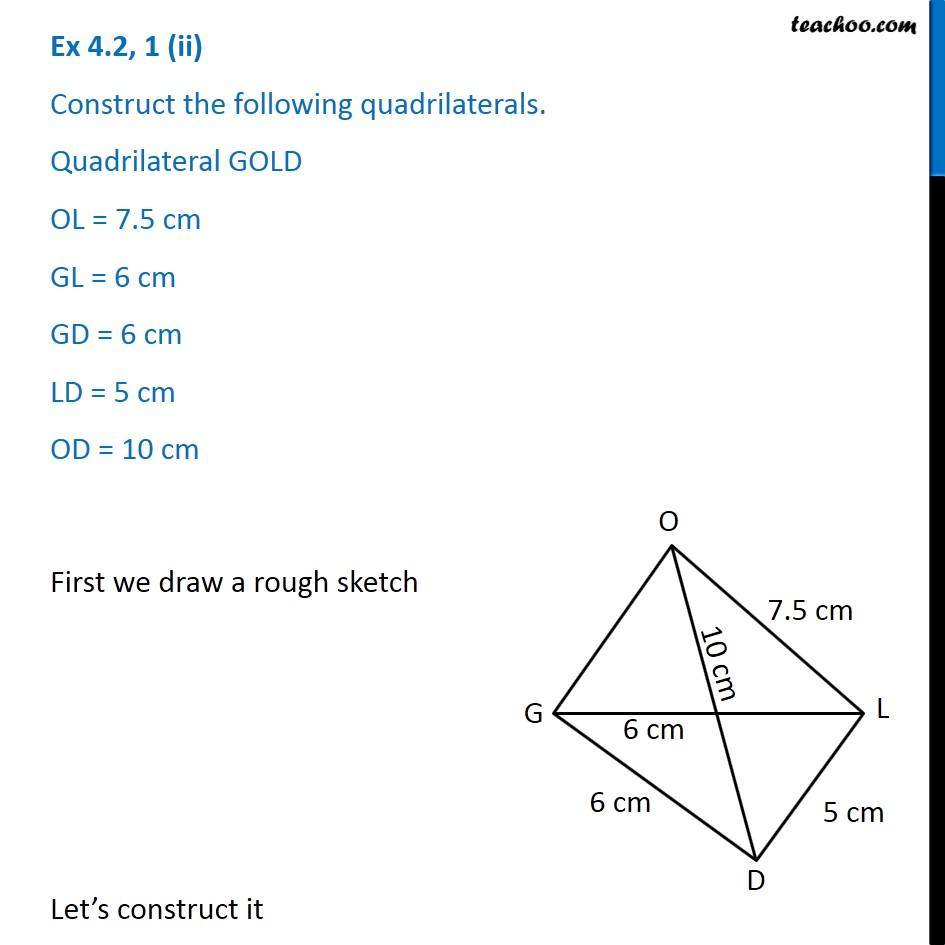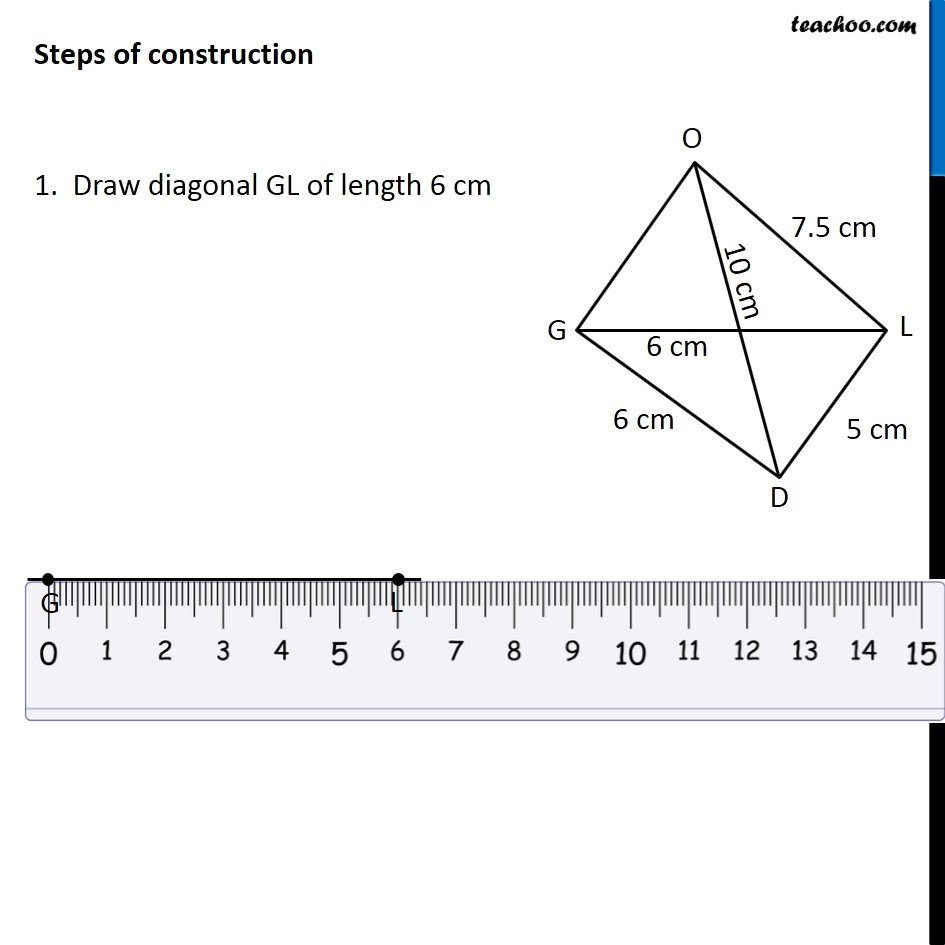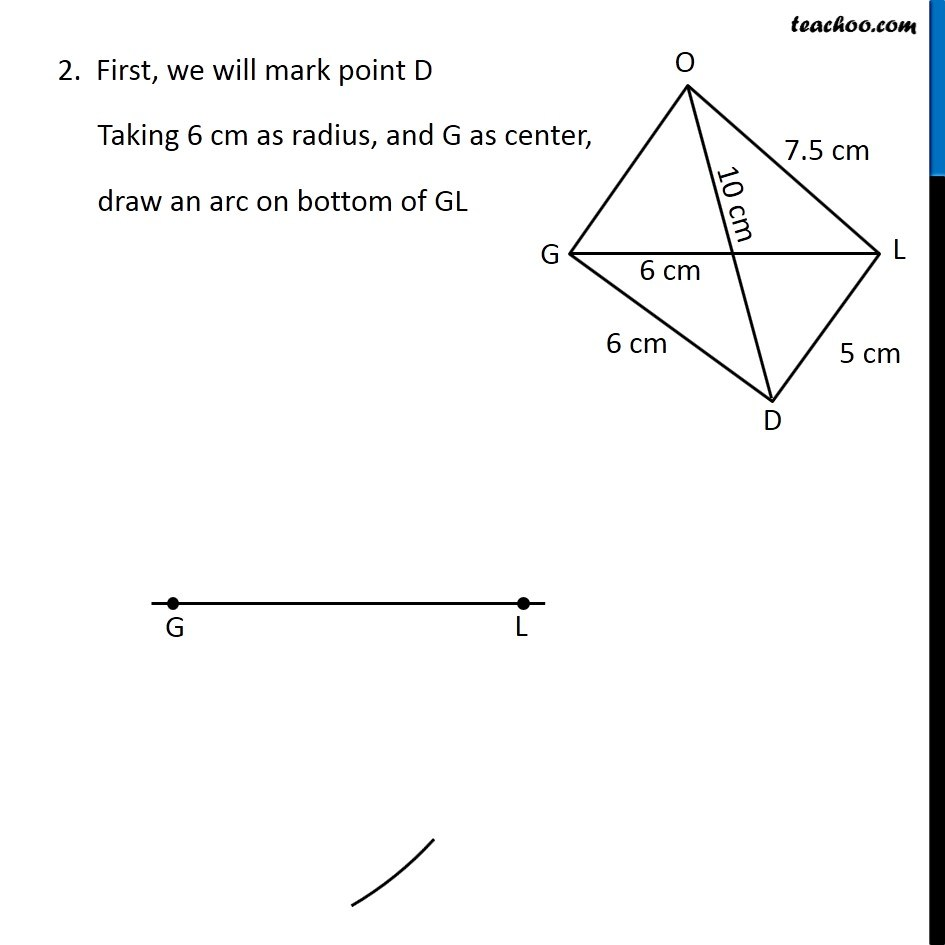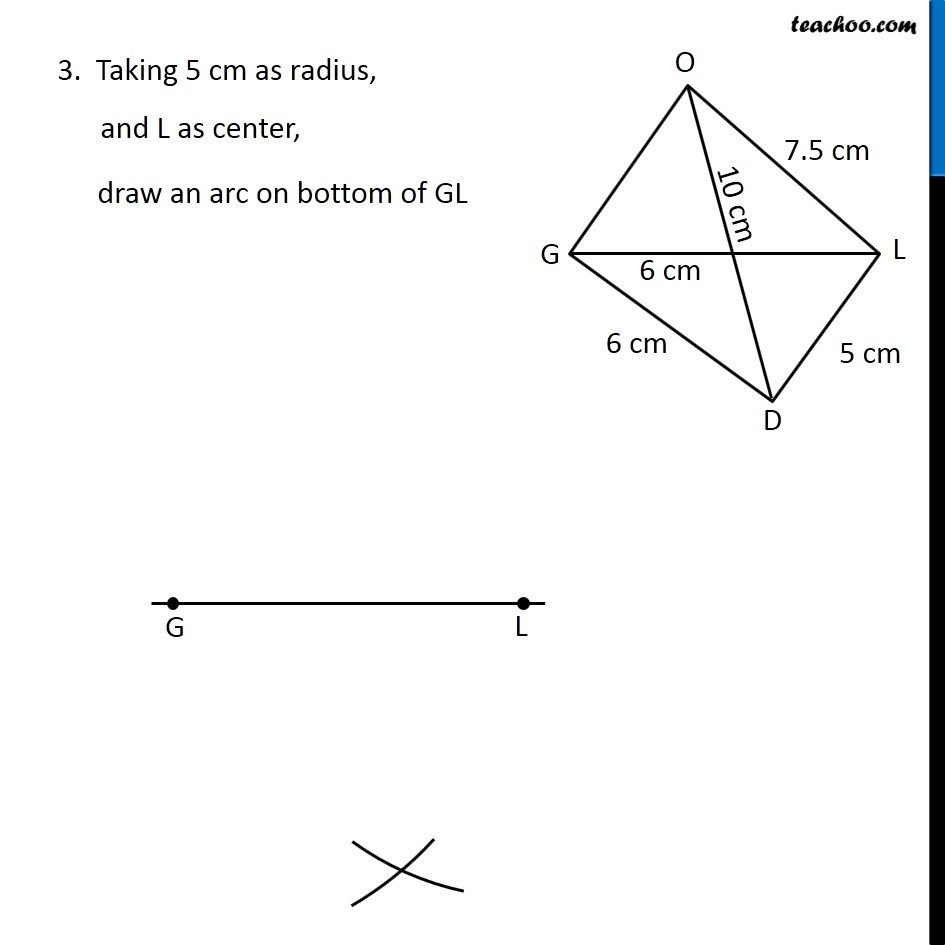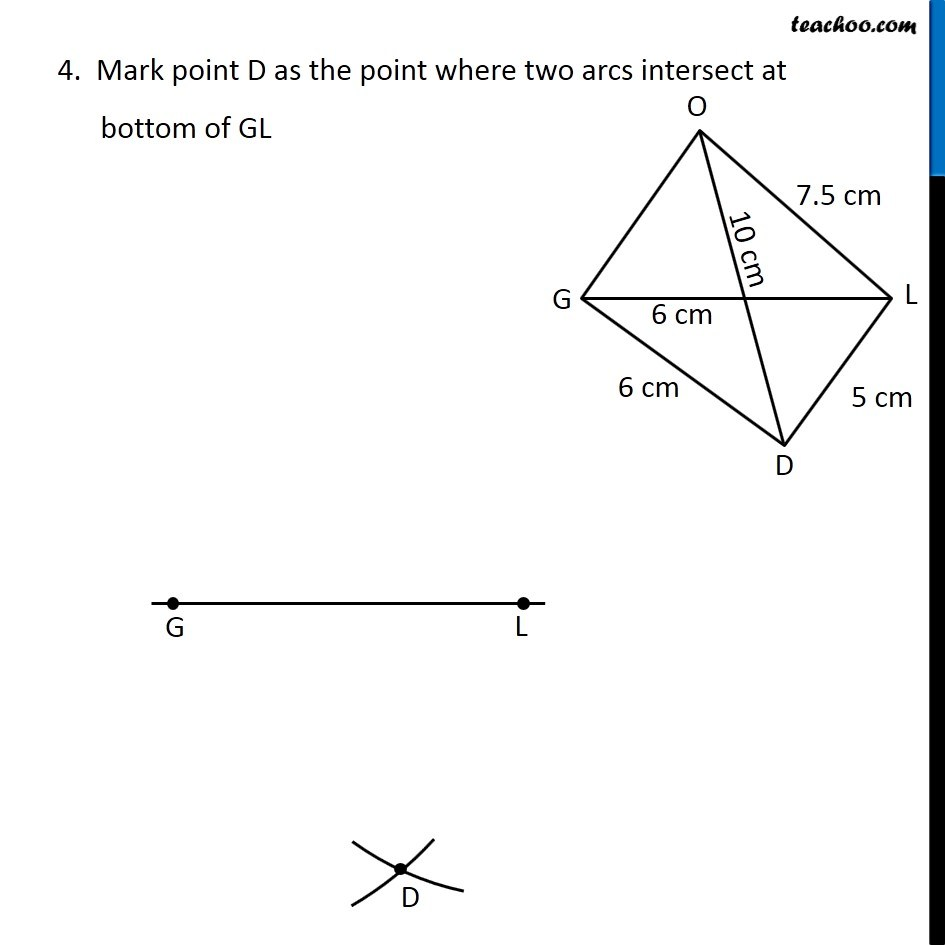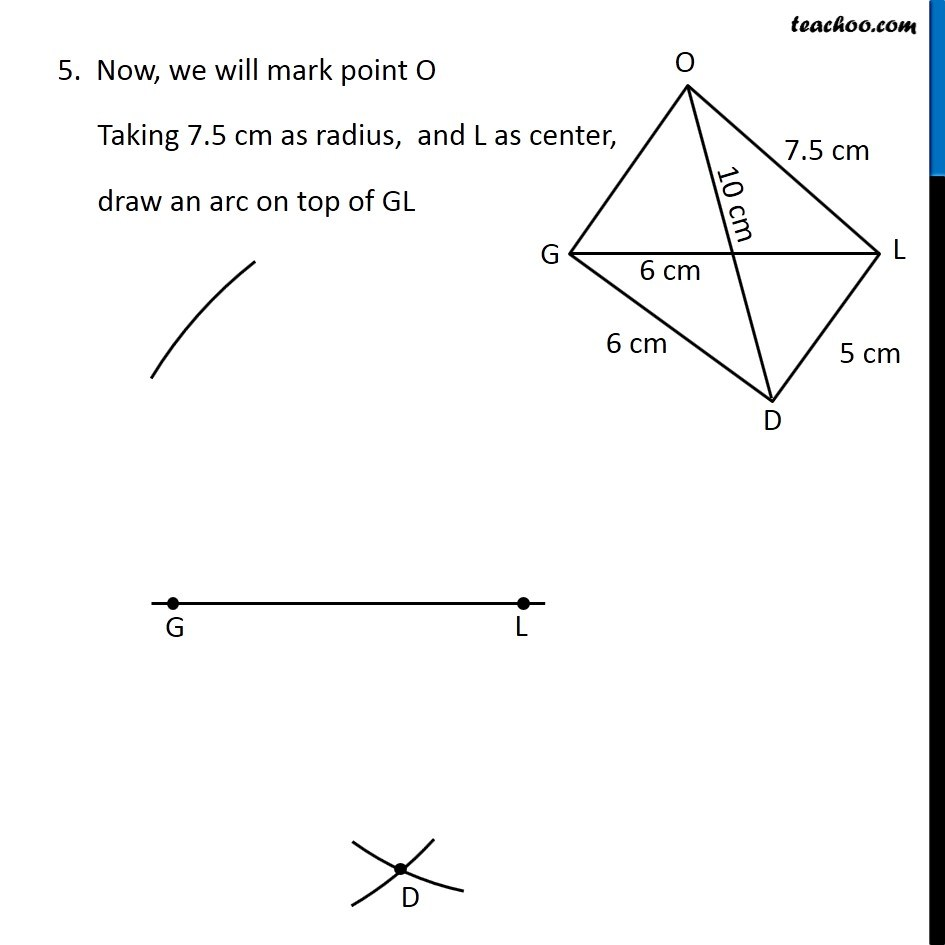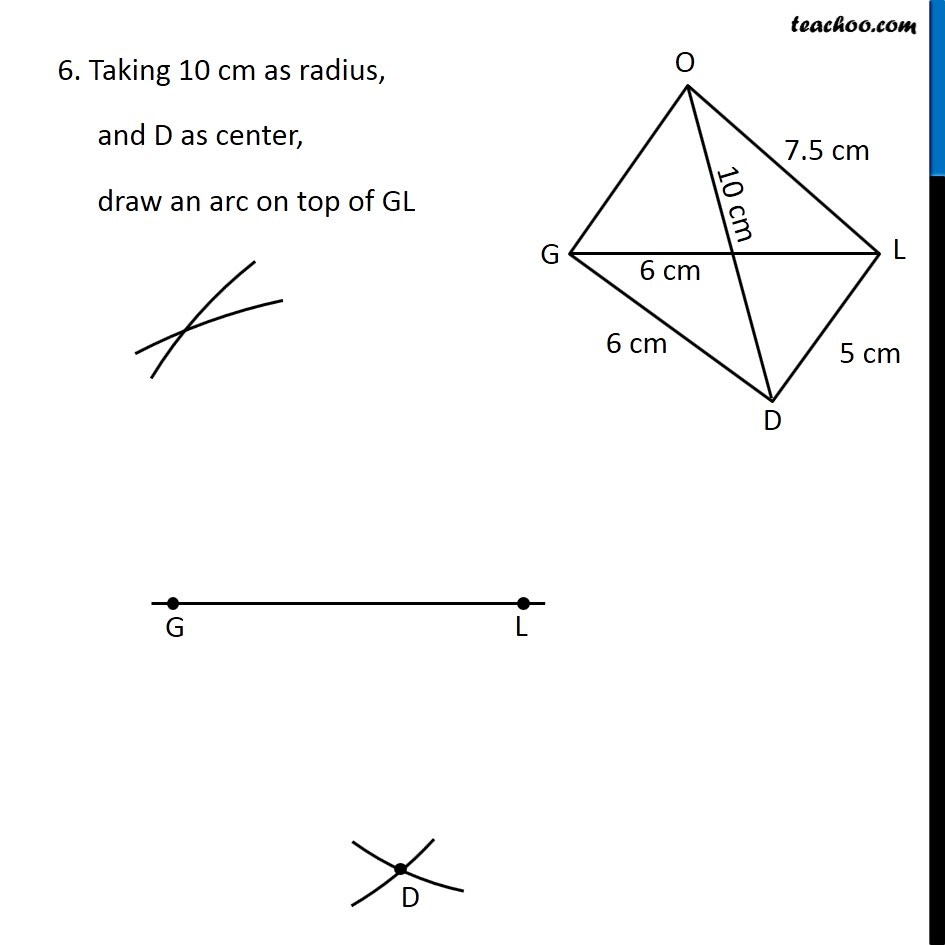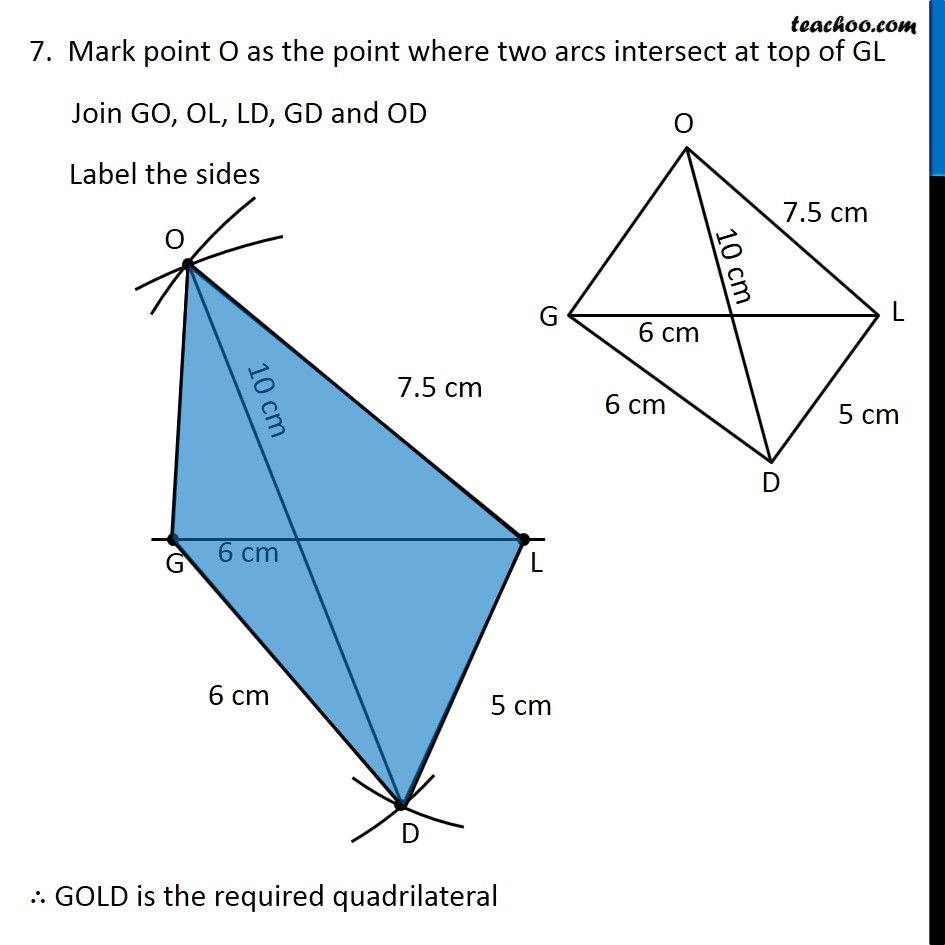Get live Maths 1-on-1 Classs - Class 6 to 12

### Transcript

Ex 4.2, 1 (ii) Construct the following quadrilaterals. Quadrilateral GOLD OL = 7.5 cm GL = 6 cm GD = 6 cm LD = 5 cm OD = 10 cm First we draw a rough sketch Let’s construct it Steps of construction 1. Draw diagonal GL of length 6 cm 2. First, we will mark point D Taking 6 cm as radius, and G as center, draw an arc on bottom of GL 3. Taking 5 cm as radius, and L as center, draw an arc on bottom of GL 4. Mark point D as the point where two arcs intersect at bottom of GL 5. Now, we will mark point O Taking 7.5 cm as radius, and L as center, draw an arc on top of GL 6. Taking 10 cm as radius, and D as center, draw an arc on top of GL 7. Mark point O as the point where two arcs intersect at top of GL Join GO, OL, LD, GD and OD Label the sides